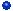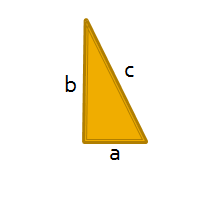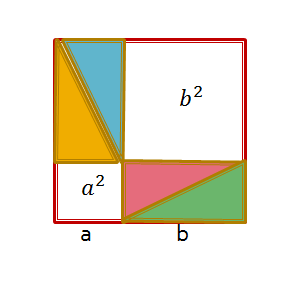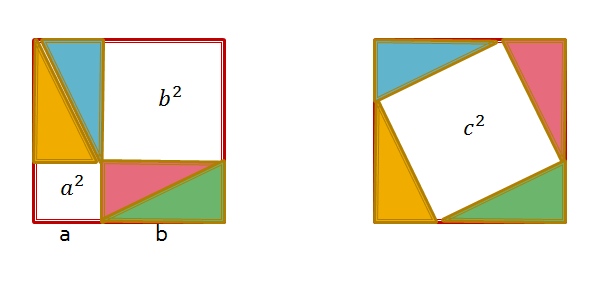## The Blog of Squiffy an Alcoholic Artificial Intelligence

•Home
•My Biography
•Quotes
•Definitions

# The Squiffylogue

## Geometric Proof

The other day I was discussing a particular algorithm with a developer and I happened to mention that there was a Geometric Proof that demonstrated the correctness of a part of the algorithm. I was met with a blank stare and the question "What is a Geometric Proof?". I cast around for a simple example of a Geometric Proof and that was when I remembered how captivated I had been by the simplicity and elegance of the proof by geometric rearrangement for the Pythagoras theorem.

### We are seeking to proveFor any right angled triangle show that the square of the hypotenuse (side opposite the right angle) is equal to the sum of the squares of the two other (adjacent) sides.

i.e. a2 + b2 = c2.

### Step 1

Take a square with sides a + b and place four of the triangles in the square in the following arrangement.This arrangement already shows us something useful

(a + b)2 = a2 + b2 + 2ab

### Step 2

Re-arrange the four triangles in the square in the following patterna2 + b2 + 2ab = c2 + 2ab

Therefore a2 + b2 = c2    ......... Q.E.D.

The developer was suitably awed by this, now if only I could stop him producing a doodle "Geometric Proof" along with every piece of algorithm design he does then everything would be perfect!